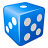# Label script cutting off last character?

2006
3
03-07-2012 07:26 AMNew Contributor
Ok, so I don't know that much about vbScripts, but I am using this one to make the text on the label concatenate to fields into the label, wrap the text at a certain number of characters and insert a new line.

Function FindLabel ( [NAME], [PARKING_SP] )
FindLabel = " [NAME]  & [PARKING_SP] "

Dim finalLabel, strInput, curChar, PWS, CWS
Dim c, numChars, senLimit, numSpaces, spaceIter, CWSLen
strInput =  [NAME]  & vbNewLine &  [PARKING_SP]  '* label field names NAME & PARKING_SP
intInput = [PARKING_SP]
senLimit = 15 '*sentence limit is 15

finalLabel = ""
strLabel = ""
intLabel = ""

numChars = Len(strInput)

'Determine number of spaces in string
numSpaces = 0
c = 0
Do Until (c = numChars)
c = c + 1
curChar = Mid(strInput, c, 1)
If (curChar = " ") Then
numSpaces = numSpaces + 1
End If
Loop

c=0
Do Until (spaceIter = numSpaces + 1)
Do Until (curChar = " ") Or ((c + 1) > numChars)
c = c + 1
curChar = Mid(strInput, c, 1)
CWS = CWS + curChar
Loop
CWSLen = Len(CWS)
If (CWSLen > senLimit) Then
PWS = Left(CWS, (CWSLen - 1))
PWSLen = Len(PWS)
If (Len(PWS) > senLimit) Then
Do Until (Right(PWS, 1) = " ")
PWS = Left(PWS, PWSLen)
PWSLen = PWSLen - 1
c = c - 1
Loop
End If
curChar = "dummy"
PWS = Trim(PWS)
If (finalLabel <> "") Then
finalLabel = finalLabel & vbNewLine & PWS
Else
finalLabel = PWS
End If
CWS = ""
spaceIter = spaceIter - 1
Else
If ((c + 1) <= numChars) Then
PWS = Left(CWS, (CWSLen - 1))
curChar = "dummy"
End If
spaceIter = spaceIter + 1
End If
Loop

If (finalLabel <> "") Then
finalLabel = finalLabel & vbNewLine & CWS
Else
finalLabel = cws
End If

FindLabel = finalLabel

End Function

The problem is that it is cutting off the final character in the last line that is pulling in the number of parking spaces, but only on
*some* of the labels, not all of them....help!
Tags (2)
3 RepliesOccasional Contributor III
I've had this same problem and am very interested in the solution.Occasional Contributor III
Any help on this out there?by Anonymous User
Not applicable
I took a stab at this...Very tricky! (or maybe I am just over thinking?) I was able to generate the labels properly using Python and printing out the labels using a search cursor, but I was unable to get this to work as a label expression. It is also very clunky. Hopefully someone who is more experienced with writing label expressions can get this to work.

```def FindLabel(field,length):
wlist = field.split(" ")
linelist = []
scratch = []
chars = ""
for w in wlist:
if len(chars) <= length:
chars += " ".join(["",w])
chars = chars.lstrip()

if len(chars) > length:
linelist.append(" ".join(i for i in chars.split(" ")[:-1]))
chars = chars.split(" ")[-1]

if len(chars) < length:
pass
elif len(chars) == length:
linelist.append(chars)
else:
linelist.append(chars)
else:
scratch.append(chars)
try:
if scratch[-1] not in linelist:
linelist.append(scratch[-1])
if wlist[-1] not in linelist:
linelist.append(wlist[-1])
if linelist[-2].split(" ")[-1] == linelist[-1]:
del linelist[-1]
except:
pass

label = "\n".join(str(i) for i in linelist)
return label

if __name__ == '__main__':  # Cut this out of label expression

import arcpy

dbf = r'C:\Testing\deed.dbf'
with arcpy.da.SearchCursor(dbf,['DEEDHOLDER']) as rows:
for row in rows:
name = row
label = FindLabel(name,15)
print label
if label.replace("\n"," ") == name:
print '\ntrue\n'
else:
print '\nfalse\n'

```

I was able to successfully get this to wrap texts when printing out to the interactive python window, but getting this to work as a label expression is a whole new ball game. I tested many different lengths (10, 15, 20, 25, 30) and it properly wrapped the text. I also did a test to make sure that all the values in the field match up after generating the labels and they all returned true.

[ATTACH=CONFIG]22963[/ATTACH]